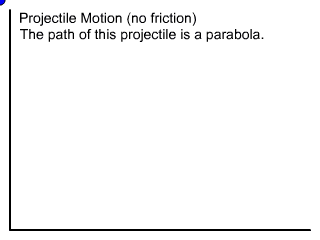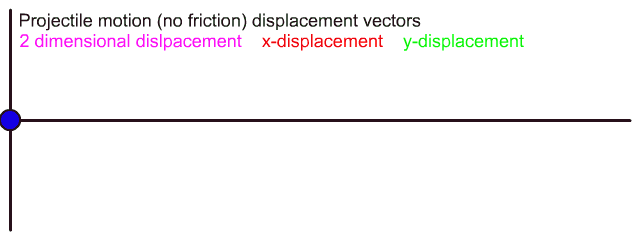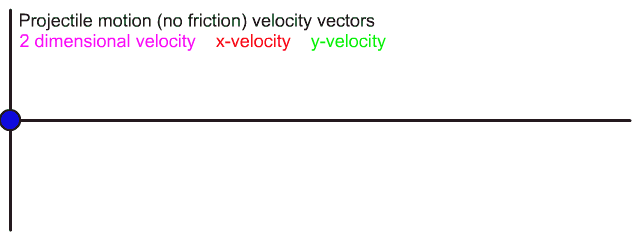﻿ Projectile Motion | Zona Land Education

# Projectile Motion

 Your browser does not support inline frames or is currently configured not to display inline frames. Click the picture to the left. Intro below, other topics:

What is projectile motion?Here we will define a projectile as a moving object that is thrown above and across ground near the surface of the Earth. The path of the projectile is called its trajectory.

In most of our discussions here we will consider projectile motion to be without air resistance. For small rock-like objects hand tossed at moderate velocities air resistance is small and can be ignored without generating too much error. Under these conditions of no air resistance, the path of a projectile is a parabola.

If the object has a lot of air resistance, say, like a loosely crumpled piece of tissue paper, then its motion will be more complicated than the zero air resistance explanations shown here.

Where is the projectile?The projectile is thrown from its starting location which is called its initial position. As it moves the projectile is displaced both horizontally and vertically from this initial position.

The two dimensional displacement is a vector that has a length equal to the distance from the initial position to the current location of the projectile. The angle for this vector is usually stated relative to the horizontal. This two dimensional displacement vector is shown in this color in the above animation.

The two dimensional displacement has x- and y-components. The x-displacement marks the current horizontal position, and the y-displacement marks the current vertical position. The x-displacement is shown in this color, and the y-displacement in this color.

How fast is the projectile traveling and where is it headed?The velocity vector for the projectile marks its speed and direction.

In the above two dimensional animation the two dimensional velocity vector is shown in this color. This vector has x- and y-components.

The x-velocity describes how the projectile is moving across ground, or down range. It is this color. Note that the x-velocity does not change size. The speed of the projectile stays constant horizontally. Since there is no change in the x-velocity, there is no x-acceleration. This is because there are no x-directed forces. Gravity does not pull sideways.

The y-velocity describes how the object is moving up or down, and it is drawn in this color. Note that the y-velocity is not constant. Its size gets smaller on the way up, its size is zero at the top, and its size grows on the way down. Since there is a change in the y-velocity, there is a y-acceleration. This is because there is a y-directed force: gravity.

Custom Search